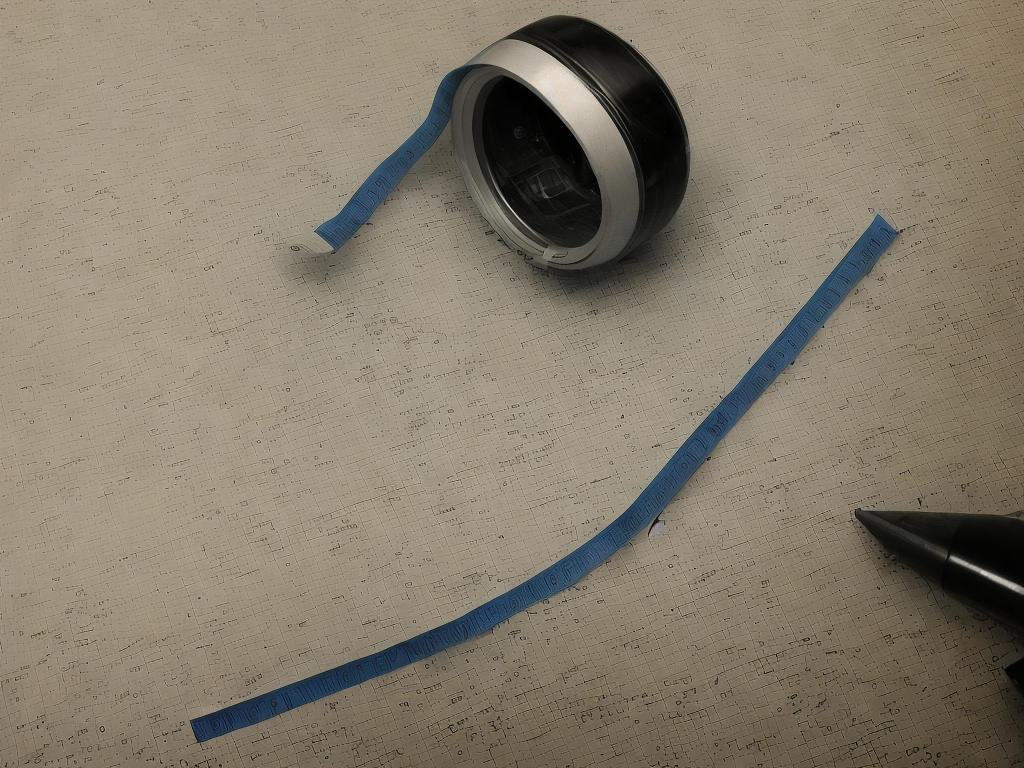# Difference Between Relative And Percentage Error

tl;dr
Relative error is the ratio of the difference between the measured value and the actual value to the actual value, while percentage error is the difference between the measured value and the actual value expressed as a percentage of the actual value.In the world of mathematics and science, accuracy is an essential component. When calculating values, there are bound to be errors. These errors can be caused by a variety of reasons such as human error, limitation of measurement devices, or environmental factors. As a result, two common ways of describing the accuracy of a measurement are percentage error and relative error. This article aims to explore the difference between these two concepts.

What is Relative Error?

Relative error is a measure of the error of a measured quantity compared to its actual value. It is defined as the ratio of the difference between the measured value and the actual value to the actual value. Mathematically, it is given by the formula:

Relative Error = (Measured Value - Actual Value) / Actual Value

For example, suppose we want to calculate the relative error of measuring a piece of wood 8.5 cm long, but the actual length is 8 cm. Here, the measured value is 8.5 cm, and the actual value is 8 cm. Therefore, relative error would be calculated as:

Relative Error = (Measured Value - Actual Value) / Actual Value

Relative Error = (8.5 - 8) / 8

Relative Error = 0.0625 or 6.25%

The relative error in this example is 6.25%, which tells us that the measured value is 6.25% higher than the actual value.

What is Percentage Error?

Percentage error, also known as percent error, is another measure of accuracy. It indicates the difference between the actual value and the recorded value expressed as a percentage of the actual value. The formula for percentage error is:

Percentage Error = (|Measuered Value - Actual Value| / Actual Value) x 100

It is worth noting that the absolute value of the difference between the actual value and the measured value is taken to ensure that the result is positive.

For example, let's take an example of measuring the length of a table. Suppose the actual length of the table is 120 cm, and we measure it to be 118 cm. Then the percentage error can be calculated as:

Percentage Error = (|118 - 120| / 120) x 100

Percentage Error = (2 / 120) x 100

Percentage Error = 1.67%

The percentage error in this case is 1.67%, indicating an error of 1.67% over the actual value.

Difference between Relative Error and Percentage Error

The primary difference between relative error and percentage error lies in the method of calculation. While Relative error is calculated as a ratio of the difference between the measured and actual values to the actual value, percentage error is calculated as a percentage of the error compared to the actual value. However, both these measures of errors are used to gauge the level of accuracy of the measurement, but it ultimately depends on what the situation calls for.

Another key difference between the two concepts is the range of values that they can have. Relative error can be any value between negative infinity and infinity. On the other hand, percentage error always ranges from 0 to 100%. This is because it is expressed as a percentage, which cannot be negative or greater than 100%.

Both these forms of errors are useful to understand the accuracy of a measured value. Still, the choice between the two depends on the scale of the measurements and the application for which the measurements are being made. For instance, in the case of extremely small values like measuring the mass of the atom or electron, relative error is a more appropriate measure. In contrast, in scenarios such as calibrating scales or measuring the weight of merchandise, percentage error is preferred.

Conclusion

To summarize, both relative and percentage errors are measures of accuracy and can be used interchangeably but depend on the scale and type of measurement. It is essential to understand that errors in measurements are inevitable, but it is the job of scientists and researchers to ensure that the errors do not impact the results significantly. Correctly assessing errors is crucial to producing accurate results, and both percentage and relative errors provide us with invaluable insight into the level of accuracy of our measurements.